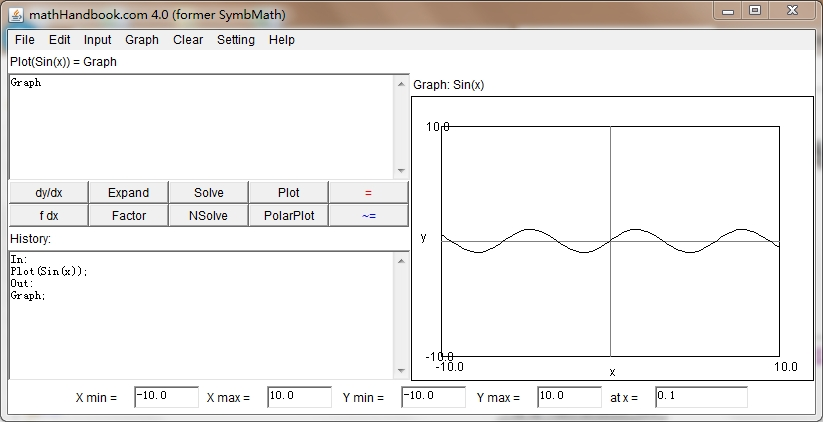﻿ ﻿﻿ Fractional Calculus Computer Algebra System math software++=﻿

# Mathematics

Mathematics (colloquially, maths or math), is the body of knowledge centered on concepts such as quantity, structure, space, and change, and also the academic discipline that studies them. Benjamin Peirce called it "the science that draws necessary conclusions". Other practitioners of mathematics maintain that mathematics is the science of pattern, that mathematicians seek out patterns whether found in numbers, space, science, computers, imaginary abstractions, or elsewhere. Mathematicians explore such concepts, aiming to formulate new conjectures and establish their truth by rigorous deduction from appropriately chosen axioms and definitions.

Through the use of abstraction and logical reasoning, mathematics evolved from counting, calculation, measurement, and the systematic study of the shapes and motions of physical objects. Knowledge and use of basic mathematics have always been an inherent and integral part of individual and group life. Refinements of the basic ideas are visible in mathematical texts originating in ancient Egypt, Mesopotamia, ancient India, ancient China, and ancient Greece. Rigorous arguments first appear in Euclid's Elements. The development continued in fitful bursts until the Renaissance period of the 16th century, when mathematical innovations interacted with new scientific discoveries, leading to an acceleration in research that continues to the present day.

Today, mathematics is used throughout the world in many fields, including natural science, engineering, medicine, and the social sciences such as economics. Applied mathematics, the application of mathematics to such fields, inspires and makes use of new mathematical discoveries and sometimes leads to the development of entirely new disciplines. Mathematicians also engage in pure mathematics, or mathematics for its own sake, without having any application in mind, although applications for what began as pure mathematics are often discovered later.

see handbook

SIR model

## Book

• handBook
• math handbook (Russian)
• Handbook of Special Functions
• Graduate Texts in Mathematics (GTM) 1-280
﻿
ALGEBRAIC NUMBER THEORY - MILNE.pdf
geometric algebra for computer graphics.pdf
GTM.Probability -A.N.Shiryaev.pdf
GTM065.Differential Analysis on Complex Manifolds.pdf
GTM207.Algebraic.Graph.Theory,.Godsil,.Royle.pdf
GTM208.Analysis.for.Applied.Mathematics,.Ward.Cheney.2001.pdf
GTM211.Algebra  -Serge Lang.pdf
GTM213.From.Holomorphic.Functions.to.Complex.Manifolds,.Fritzsche.pdf
GTM274.Brownian Motion, Martingales, and Stochastic Calculus.pdf
integral table.pdf
list.txt
ordinary differential equations-Arnol'd.pdf
Oxford User's Guide to Mathematics-2004.pdf
Princeton to math.pdf
Real_Analysis_Fon-Che_Liu.pdf
Riemann Geometry.pdf


﻿